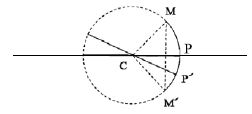Physics

# Some Definitions Related to Spherical MirrorsDefinitions Related to Spherical Mirrors:

Pole: The central point of the reflecting surface of a spherical mirror is called the pole of the mirror. In figure (1), P is the pole of the mirror. It is the midpoint of the spherical mirror. Pole is the lowest point of the reflecting surface in the case of a concave mirror, while it is the highest point in a convex mirror. The pole of a spherical mirror lies on the surface of the mirror.

Center of curvature: The center of the sphere of which the spherical minor is a part is called the center of curvature of that minor. This spherical mirror is the point in the center of the mirror which passes through the curve of the mirror and has the same tangent and curvature at that point. In figure (1), C is the center of curvature. It’s the midpoint of the spherical mirror. It is the point in the center of the mirror that passes through the curve of the mirror and has the same tangent and curvature at that point. The center of curvature of a concave mirror is in front of it and the center of curvature of a convex mirror is behind the mirrors as shown in the figure.

The radius of curvature: The radius of the sphere of which the spherical minor is a part is called the radius of curvature of that mirror. In figure (1), PC or MC is the radius of curvature. The radius of curvature of a spherical mirror is the radius of the imaginary hollow sphere of glass, of which the spherical mirror is a part. It’s the linear distance between the Pole and the Center of curvature. The radius of curvature is denoted by r. The distance PC is the radius of curvature of a convex mirror.Principal axis: The straight line passing through the pole and the center of curvature of a spherical mirror is called the principal axis of that mirror. It’s an imaginary line passing through the optical center and the center of curvature of any lens or a spherical mirror. In figure (1), PC is the principal axis. The principal axis of a spherical mirror is the straight line passing through the center of curvature C and pole P of a spherical mirror, produced on both sides.

Secondary axis: The straight line passing through the center of curvature and any point other than the pole on the reflecting surface of the sphere is called the secondary axis. In figure (1), P’C is the secondary axis.

Aperture: An aperture of a mirror or lens is a point from which the reflection of light actually happens. It also gives the size of the mirror.

Principal Focus: Principal Focus can also be called Focal Point. It’s on the axis of a mirror or lens wherein rays of light parallel to the axis converge or appear to converge after reflection or refraction.